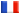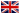# Séminaire de Cryptographie

## Oded Regev### On Lattices, Learning with Errors, Random Linear Codes, and Cryptography

Our main result is a reduction from worst-case lattice problems such as SVP and SIVP to a certain learning problem. This learning problem is a natural extension of the `learning from parity with error' problem to higher moduli. It can also be viewed as the problem of decoding from a random linear code. This, we believe, gives a strong indication that these problems are hard. Our reduction, however, is quantum. Hence, an efficient solution to the learning problem implies a _quantum_ algorithm for SVP and SIVP. A main open question is whether this reduction can be made classical.

Using the main result, we obtain a public-key cryptosystem whose hardness is based on the worst-case quantum hardness of SVP and SIVP. Previous lattice-based public-key cryptosystems such as the one by Ajtai and Dwork were only based on unique-SVP, a special case of SVP. The new cryptosystem is much more efficient than previous cryptosystems: the public key is of size \tilde{O}(n) and encrypting a message increases its size by \tilde{O}(n) (in previous cryptosystems these values are \tilde{O}(n^4) and \tilde{O}(n^2), respectively).